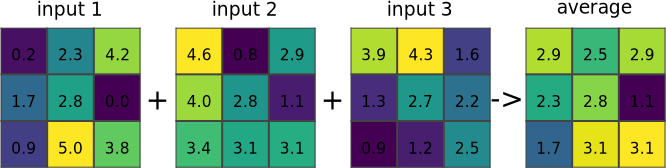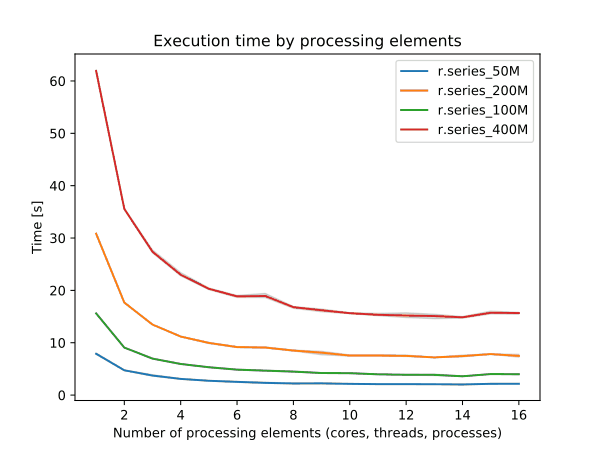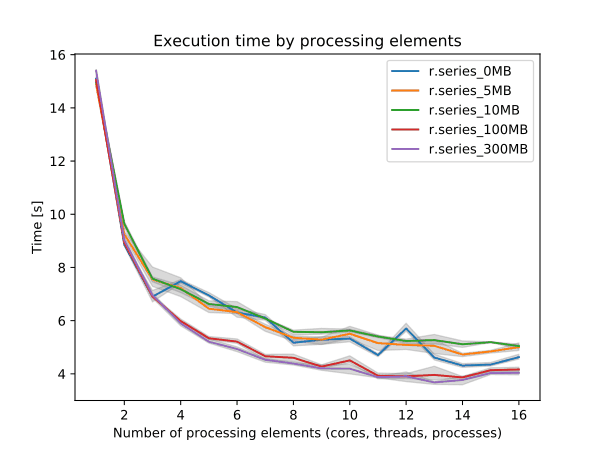## NAME

r.series - Makes each output cell value a function of the values assigned to the corresponding cells in the input raster map layers.

## KEYWORDS

raster, aggregation, series, parallel

## SYNOPSIS

r.series
r.series --help
r.series [-nz] [input=name[,name,...]] [file=name] output=name[,name,...] method=string[,string,...] [quantile=float[,float,...]] [weights=float[,float,...]] [range=lo,hi] [nprocs=integer] [memory=memory in MB] [--overwrite] [--help] [--verbose] [--quiet] [--ui]

### Flags:

-n
Propagate NULLs
-z
Do not keep files open
--overwrite
Allow output files to overwrite existing files
--help
Print usage summary
--verbose
Verbose module output
--quiet
Quiet module output
--ui
Force launching GUI dialog

### Parameters:

input=name[,name,...]
Name of input raster map(s)
file=name
Input file with one raster map name and optional one weight per line, field separator between name and weight is |
output=name[,name,...] [required]
Name for output raster map
method=string[,string,...] [required]
Aggregate operation
Options: average, count, median, mode, minimum, min_raster, maximum, max_raster, stddev, range, sum, variance, diversity, slope, offset, detcoeff, tvalue, quart1, quart3, perc90, quantile, skewness, kurtosis
quantile=float[,float,...]
Quantile to calculate for method=quantile
Options: 0.0-1.0
weights=float[,float,...]
Weighting factor for each input map, default value is 1.0 for each input map
range=lo,hi
Ignore values outside this range
nprocs=integer
Number of threads for parallel computing
Default: 1
memory=memory in MB
Maximum memory to be used (in MB)
Cache size for raster rows
Default: 300

## DESCRIPTION

r.series makes each output cell value a function of the values assigned to the corresponding cells in the input raster map layers.Figure: Illustration for an average of three input rasters

Following methods are available:

• average: average value
• count: count of non-NULL cells
• median: median value
• mode: most frequently occurring value
• minimum: lowest value
• min_raster: raster map number with the minimum time-series value
• maximum: highest value
• max_raster: raster map number with the maximum time-series value
• stddev: standard deviation
• range: range of values (max - min)
• sum: sum of values
• variance: statistical variance
• diversity: number of different values
• slope: linear regression slope
• offset: linear regression offset
• detcoeff: linear regression coefficient of determination
• tvalue: linear regression t-value
• quart1: first quartile
• quart3: third quartile
• perc90: ninetieth percentile
• quantile: arbitrary quantile
• skewness: skewness
• kurtosis: kurtosis
Note that most parameters accept multiple answers, allowing multiple aggregates to be computed in a single run, e.g.:

```r.series input=map1,...,mapN \
output=map.mean,map.stddev \
method=average,stddev
```
or:

```r.series input=map1,...,mapN \
output=map.p10,map.p50,map.p90 \
method=quantile,quantile,quantile \
quantile=0.1,0.5,0.9
```
The same number of values must be provided for all options.

## NOTES

### No-data (NULL) handling

With -n flag, any cell for which any of the corresponding input cells are NULL is automatically set to NULL (NULL propagation). The aggregate function is not called, so all methods behave this way with respect to the -n flag.

Without -n flag, the complete list of inputs for each cell (including NULLs) is passed to the aggregate function. Individual aggregates can handle data as they choose. Mostly, they just compute the aggregate over the non-NULL values, producing a NULL result only if all inputs are NULL.

### Minimum and maximum analysis

The min_raster and max_raster methods generate a map with the number of the raster map that holds the minimum/maximum value of the time-series. The numbering starts at 0 up to n for the first and the last raster listed in input=, respectively.

### Range analysis

If the range= option is given, any values which fall outside that range will be treated as if they were NULL. The range parameter can be set to low,high thresholds: values outside of this range are treated as NULL (i.e., they will be ignored by most aggregates, or will cause the result to be NULL if -n is given). The low,high thresholds are floating point, so use -inf or inf for a single threshold (e.g., range=0,inf to ignore negative values, or range=-inf,-200.4 to ignore values above -200.4).

### Linear regression

Linear regression (slope, offset, coefficient of determination, t-value) assumes equal time intervals. If the data have irregular time intervals, NULL raster maps can be inserted into time series to make time intervals equal (see example).

### Quantiles

r.series can calculate arbitrary quantiles.

### Memory consumption

Memory usage is not an issue, as r.series only needs to hold one row from each map at a time.

### Management of open file limits

The maximum number of raster maps that can be processed is given by the user-specific limit of the operating system. For example, the soft limits for users are typically 1024 files. The soft limit can be changed with e.g. ulimit -n 4096 (UNIX-based operating systems) but it cannot be higher than the hard limit. If the latter is too low, you can as superuser add an entry in:
```/etc/security/limits.conf
# <domain>      <type>  <item>         <value>
```
This will raise the hard limit to 4096 files. Also have a look at the overall limit of the operating system
```cat /proc/sys/fs/file-max
```
which on modern Linux systems is several 100,000 files.

For each map a weighting factor can be specified using the weights option. Using weights can be meaningful when computing the sum or average of maps with different temporal extent. The default weight is 1.0. The number of weights must be identical to the number of input maps and must have the same order. Weights can also be specified in the input file.

Use the -z flag to analyze large amounts of raster maps without hitting open files limit and the file option to avoid hitting the size limit of command line arguments. Note that the computation using the file option is slower than with the input option. For every single row in the output map(s) all input maps are opened and closed. The amount of RAM will rise linearly with the number of specified input maps. The input and file options are mutually exclusive: the former is a comma separated list of raster map names and the latter is a text file with a new line separated list of raster map names and optional weights. As separator between the map name and the weight the character "|" must be used.

### Performance

To enable parallel processing, the user can specify the number of threads to be used with the nprocs parameter (default 1). The memory parameter (default 300 MB) can also be provided to determine the size of the buffer in MB for computation.Figure: Benchmark on the left shows execution time for different number of cells, benchmark on the right shows execution time for different memory size for 10000x10000 raster. See benchmark scripts in source code. (Intel Core i9-10940X CPU @ 3.30GHz x 28)

To reduce the memory requirements to minimum, set option memory to zero. To take advantage of the parallelization, GRASS GIS needs to compiled with OpenMP enabled.

## EXAMPLES

Using r.series with wildcards:
```r.series input="`g.list pattern='insitu_data.*' sep=,`" \
output=insitu_data.stddev method=stddev
```

Note the g.list script also supports regular expressions for selecting map names.

Using r.series with NULL raster maps (in order to consider a "complete" time series):

```r.mapcalc "dummy = null()"
r.series in=map2001,map2002,dummy,dummy,map2005,map2006,dummy,map2008 \
out=res_slope,res_offset,res_coeff meth=slope,offset,detcoeff
```

Example for multiple aggregates to be computed in one run (3 resulting aggregates from two input maps):

```r.series in=one,two out=result_avg,res_slope,result_count meth=sum,slope,count
```

Example to use the file option of r.series:

```cat > input.txt << EOF
map1
map2
map3
EOF

r.series file=input.txt out=result_sum meth=sum
```

Example to use the file option of r.series including weights. The weight 0.75 should be assigned to map2. As the other maps do not have weights we can leave it out:

```cat > input.txt << EOF
map1
map2|0.75
map3
EOF

r.series file=input.txt out=result_sum meth=sum
```

Example for counting the number of days above a certain temperature using daily average maps ('???' as DOY wildcard):

```# Approach for shell based systems
r.series input=`g.list rast pattern="temp_2003_???_avg" separator=comma` \
output=temp_2003_days_over_25deg range=25.0,100.0 method=count

# Approach in two steps (e.g., for Windows systems)
g.list rast pattern="temp_2003_???_avg" output=mapnames.txt
r.series file=mapnames.txt \
output=temp_2003_days_over_25deg range=25.0,100.0 method=count
```

g.list, g.region, r.quantile, r.series.accumulate, r.series.interp, r.univar

Glynn Clements

## SOURCE CODE

Available at: r.series source code (history)

Latest change: Monday May 16 17:29:31 2022 in commit: d207d495888a3b5c9352895e6918c46388969426

© 2003-2022 GRASS Development Team, GRASS GIS 8.3.dev Reference Manual